# Resampling Methods

## Producer Field Guide

HGD_Product
Producer Field Guide
HGD_Portfolio_Suite
Producer

The next step in the rectification and registration process is to create the output file. Since the grid of pixels in the source image rarely matches the grid for the reference image, the pixels are resampled so that new data file values for the output file can be calculated.

Resampling Process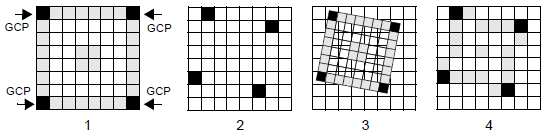In the figure above, the resampling process steps are as follows:

1 - Grid 1 shows the input image containing source GCPs.

2 - Grid 2 shows the output grid containing reference GCPs.

3 - To compare the two grids, the input image is laid over the output grid so that the GCPs of the two grids fit together.

4 - Using a resampling method, the input image pixel values are assigned to pixels in the output grid.

The following resampling methods discussed in this topic are supported in ERDAS IMAGINE:

• Nearest Neighbor—uses the value of the closest pixel to assign to the output pixel value.
• Bilinear Interpolation—uses the data file values of four pixels in a 2 × 2 window to calculate an output value with a bilinear function.
• Cubic Convolution—uses the data file values of sixteen pixels in a 4 × 4 window to calculate an output value with a cubic function.
• Bicubic Spline Interpolation—fits a cubic spline surface through the current block of points.

In all methods, the number of rows and columns of pixels in the output is calculated from the dimensions of the output map, which is determined by the geometric transformation and the cell size. The output corners (upper left and lower right) of the output file can be specified. The default values are calculated so that the entire source file is resampled to the destination file.

If an image to image rectification is being performed, it may be beneficial to specify the output corners relative to the reference file system, so that the images are coregistered. In this case, the upper left X and upper left Y coordinate are 0,0 and not the defaults.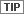If the output units are pixels, then the origin of the image is the upper left corner. Otherwise, the origin is the lower left corner.

Rectifying to Latitude Longitude

You can specify the nominal cell size if the output coordinate system is Latitude Longitude. The output cell size for a Geographic projection (that is, Latitude Longitude) is always in angular units of decimal degrees. However, if you want the cell to be a specific size in meters, you can enter meters and calculate the equivalent size in decimal degrees. For example, if you want the output file cell size to be 30 × 30 meters, then the program would calculate what this size would be in decimal degrees and automatically update the output cell size. Since the transformation between angular (decimal degrees) and nominal (meters) measurements varies across the image, the transformation is based on the center of the output file.

Enter the nominal cell size in the Nominal Cell Size dialog.

Nearest Neighbor

To determine an output pixel’s nearest neighbor, the rectified coordinates (xo, yo) of the pixel are retransformed back to the source coordinate system using the inverse of the transformation. The retransformed coordinates (xr, yr) are used in bilinear interpolation and cubic convolution as well. The pixel that is closest to the retransformed coordinates (xr, yr) is the nearest neighbor. The data file value or values for that pixel become the data file value or values of the pixel in the output image.

Nearest Neighbor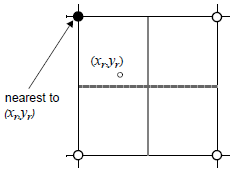Advantages Disadvantages Transfers original data values without averaging them as the other methods do; therefore, the extremes and subtleties of the data values are not lost. This is an important consideration when discriminating between vegetation types, locating an edge associated with a lineament, or determining different levels of turbidity or temperatures in a lake (Jensen, 1996). When this method is used to resample from a larger to a smaller grid size, there is usually a stair stepped effect around diagonal lines and curves. Suitable for use before classification. Data values may be dropped, while other values may be duplicated. The easiest of the three methods to compute and the fastest to use. Using on linear thematic data (for example, roads, streams) may result in breaks or gaps in a network of linear data. Appropriate for thematic files, which can have data file values based on a qualitative (nominal or ordinal) system or a quantitative (interval or ratio) system. The averaging that is performed with bilinear interpolation and cubic convolution is not suited to a qualitative class value system.

Bilinear Interpolation

In bilinear interpolation, the data file value of the rectified pixel is based upon the distances between the retransformed coordinate location (xr, yr) and the four closest pixels in the input (source) image. In the figure below, point r is the location of the retransformed coordinate. In this example, the neighbor pixels are numbered 1, 2, 3, and 4. Given the data file values of these four pixels on a grid, the task is to calculate a data file value for r (Vr).

Bilinear Interpolation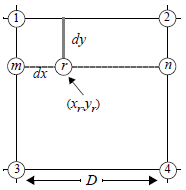To calculate Vr, first Vm and Vn are considered. By interpolating Vm and Vn, you can perform linear interpolation, which is a simple process to illustrate. If the data file values are plotted in a graph relative to their distances from one another, then a visual linear interpolation is apparent. The data file value of m (Vm) is a function of the change in the data file value between pixels 3 and 1 (that is, V3 - V1). Linear Interpolation calculates a data file value as a function of spatial distance between two pixels.

Linear Interpolation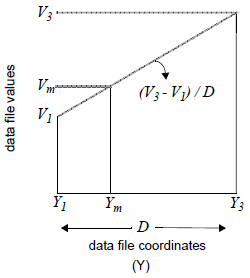The equation for calculating Vm from V1 and V3 is: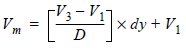Where:

Yi = Y coordinate for pixel i

Vi = data file value for pixel i

dy = distance between Y1 and Ym in the source coordinate system

D = distance between Y1 and Y3 in the source coordinate system

If one considers that (V3 - V1 / D) is the slope of the line in the graph above, then this equation translates to the equation of a line in y = mx + b form.

Similarly, the equation for calculating the data file value for n (Vn) in the pixel grid is: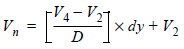From Vn and Vm, the data file value for r, which is at the retransformed coordinate location (xr,yr),can be calculated in the same manner: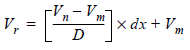The following is attained by plugging in the equations for Vm and Vn to this final equation for Vr :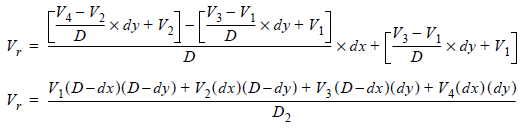In most cases D = 1, since data file coordinates are used as the source coordinates and data file coordinates increment by 1.

Some equations for bilinear interpolation express the output data file value as: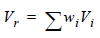Where:

wi is a weighting factor

The equation above could be expressed in a similar format, in which the calculation of wi is apparent: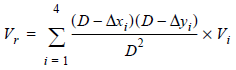Where: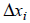= change in the X direction between (xr,yr) and the data file coordinate of pixel i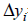= change in the Y direction between (xr,yr) and the data file coordinate of pixel i

Vi = data file value for pixel i

D = distance between pixels (in X or Y) in the source coordinate system

For each of the four pixels, the data file value is weighted more if the pixel is closer to (xr, yr).

 Advantages Disadvantages Results in output images that are smoother, without the stair-stepped effect that is possible with nearest neighbor. Since pixels are averaged, bilinear interpolation has the effect of a low-frequency convolution. Edges are smoothed, and some extremes of the data file values are lost. More spatially accurate than nearest neighbor. This method is often used when changing the cell size of the data, such as in SPOT/TM merges within the 2 × 2 resampling matrix limit.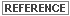See Enhancement for more about convolution filtering.

Cubic Convolution

Cubic convolution is similar to bilinear interpolation, except that:

• a set of 16 pixels, in a 4 × 4 array, are averaged to determine the output data file value, and
• an approximation of a cubic function, rather than a linear function, is applied to those 16 input values.

To identify the 16 pixels in relation to the retransformed coordinate (xr,yr), the pixel (i,j) is used, such that:

i = int (xr)

j = int (yr)

This assumes that (xr,yr) is expressed in data file coordinates (pixels). The pixels around (i,j) make up a 4 × 4 grid of input pixels, as illustrated in the following figure.

Cubic Convolution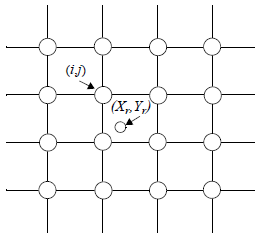Since a cubic, rather than a linear, function is used to weight the 16 input pixels, the pixels farther from (xr, yr) have exponentially less weight than those closer to (xr, yr).

Several versions of the cubic convolution equation are used in the field. Different equations have different effects upon the output data file values. Some convolutions may have more of the effect of a low-frequency filter (like bilinear interpolation), serving to average and smooth the values. Others may tend to sharpen the image, like a high-frequency filter. The cubic convolution used in ERDAS IMAGINE is a compromise between low-frequency and high-frequency. The general effect of the cubic convolution depends upon the data.

The formula used in ERDAS IMAGINE is: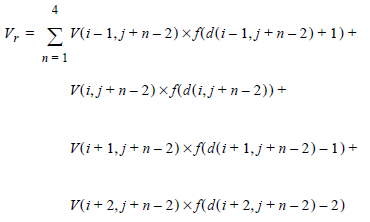Where:

i = int (xr)

j = int (yr)

d(i,j) = distance between a pixel with coordinates (i,j) and (xr,yr)

V(i,j) = data file value of pixel (i,j)

Vr = output data file value

a = -1 (a constant)

f(x) = following function: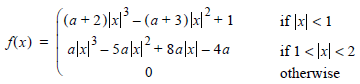Source: Atkinson, 1985

 Advantages Disadvantages Uses 4 × 4 resampling. In most cases, the mean and standard deviation of the output pixels match the mean and standard deviation of the input pixels more closely than any other resampling method. Data values may be altered. The effect of the cubic curve weighting can both sharpen the image and smooth out noise (Atkinson, 1985). The actual effects depend upon the data being used. This method is extremely slow. This method is recommended when you are dramatically changing the cell size of the data, such as in TM and aerial photo merges (that is, matches the 4 × 4 window more closely than the 2 × 2 window).

Bicubic Spline Interpolation

Bicubic Spline Interpolation is based on fitting a cubic spline surface through the current block of points. The output value is derived from the fitting surface that will retain the values of the known points. This algorithm is much slower than other methods of interpolation, but it has the advantage of giving a more exact fit to the curve without the oscillations that other interpolation methods can create. Bicubic Spline Interpolation is so similar to Bilinear Interpolation that unless you have the need to maximize surface smoothness, you should use Bilinear Interpolation.

Data Points

The known data points are an array of raster of m × n: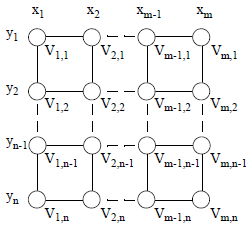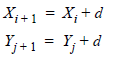Where:

1 < i < m

1 < j < n

d = cell size of the raster

Vi,j = cell value in (xi,yj)

Equations

A bicubic polynomial function V(x,y) is constructed as follows: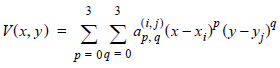in each cell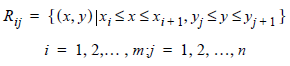• The functions and their first and second derivatives must be continuous across the interval and equal at the endpoints and the fourth derivatives of the equations should be zero.
• The function satisfies the conditions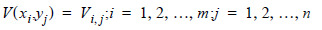that is, the spline must interpolate all data points.

• Coefficients can be obtained by resolving the known points together with the selection of the boundary condition type. See Shikin and Plis (Shikin and Plis, 1995) for the boundary conditions and the mathematical details for solving the equations. IMAGINE uses the first type of boundary condition. Because in IMAGINE the input raster grid has been expanded two cells around the boundary, the boundary condition has no significant effects on the resampling.

Calculate value for unknown point

The value for point (xr, yr) can be calculated by the following formula: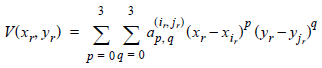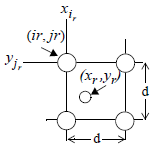The value is determined by 16 coefficients. Because the coefficients are resolved by using all other known points, all other points contribute to the value. The nearer points contribute more whereas the farther points contribute less.

 Advantages Disadvantages Results in the smoothest output images. Most computationally intensive resampling method, and is therefore the slowest. More spatially accurate than nearest neighbor. This method is often used when upsampling.

Source: Shikin and Plis, 1995